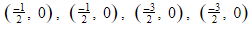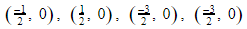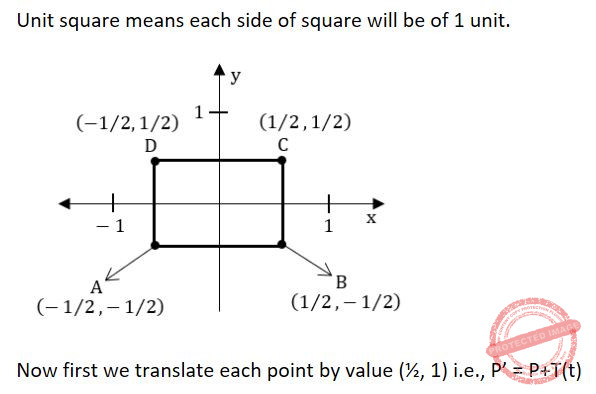## Co-ordinate-Geometry

 Question 1
Let R be the radius of the circle. What is the angle subtended by an arc of length at the center of the circle?
 A 1 degree B 1 radian C 90 degrees D π radians
Engineering-Mathematics       Co-ordinate-Geometry       ISRO CS 2014
Question 1 Explanation:
A degree (in full, a degree of arc, arc degree, or arc degree), usually denoted by ° (the degree symbol), is a measurement of a plane angle, defined so that a full rotation is 360 degrees.
It is not an SI unit, as the SI unit of angular measure is the radian, but it is mentioned in the SI brochure as an accepted unit.Because a full rotation equals 2π radians.
The radian (SI symbol rad) is the SI unit for measuring angles, and is the standard unit of angular measure used in many areas of mathematics. The length of an arc of a unit circle is numerically equal to the measurement in radians of the angle that it subtends;
 Question 2

What is the area bounded by the parabola 2y = x2 and the line x = y - 4?

 A 18 B 36 C 72 D 6
Engineering-Mathematics       Co-ordinate-Geometry       JT(IT) 2018 PART-B Computer Science
Question 2 Explanation:
Given parabola 2y = x2 --- (1)
and the line x = y – 4 ------ (2)
Then y = x+4
Now, Substitute Y value in Equation-(1)
x2 = 2 ( x + 4 ) ------ (3)
Solving equation-3 we get x = 4, - 2.
Place Values of x in equation (1) and (2) we will get y = 8, 2.
Then the points of intersection are (8, 4), (2, –2).After solving the integration, we will get 18.
 Question 3

A straight line which cuts a curve on two points at an infinite distance from the origin and yet is not itself wholly at infinity is called:

 A Spiral B Asymptote C Parallel D Polar
Engineering-Mathematics       Co-ordinate-Geometry       JT(IT) 2016 PART-B Computer Science
Question 3 Explanation:
A straight line which cuts a curve in two points at an infinite distance from the origin, but which is not itself wholly at infinity, is called an asymptote to a curve.
 Question 4
On the parabola y = x 2 find the point N least distant from the straight line y = 2 x − 4
 A N (2, 4 ) B N (1, 1 ) C N (1, 2 ) D N (− 1 , 1 )
Engineering-Mathematics       Co-ordinate-Geometry       NIELIT Junior Teachnical Assistant_2016_march
 Question 5
Three lines 3 x + y − 2 = 0 , p x + 2 y − 3 = 0 and 2 x − y − 3 = 0 intersect at a point. Then, the value of p is
 A -7 B 2 C 5 D -11
Engineering-Mathematics       Co-ordinate-Geometry       NIELIT Junior Teachnical Assistant_2016_march
 Question 6
The equation of the plane through the point ( − 1 , 3 , 2 ) and perpendicular to each of the planes x + 2 y + 3 z = 5 and 3 x + 3 y + z = 0 is
 A 7 x − 8 y + 3 z + 2 5 = 0 B 7 x + 8 y + 3 z + 2 5 = 0 C 7 x − 8 y + 3 z − 2 5 = 0 D 7 x − 8 y − 3 z − 2 5 = 0
Engineering-Mathematics       Co-ordinate-Geometry       NIELIT Junior Teachnical Assistant_2016_march
 Question 7
For the curve y = x e x the point
 A x = − 1 is a minimum B x = 0 is a minimum C x = − 1 is a maximum D x = 0 is a maximum
Engineering-Mathematics       Co-ordinate-Geometry       NIELIT Junior Teachnical Assistant_2016_march
 Question 8
The line y = m x + c will be tangent to the ellipse x 2/ 9 + y 2 / 4 = 1 , if c is equal to
 A 3 / m B √ 9m 2 + 4 C √ 1 + m 2 D √ 4m 2 + 9
Engineering-Mathematics       Co-ordinate-Geometry       NIELIT Technical Assistant_2016_march
 Question 9
The circle x 2 + y 2 + 2 ax + 1 = 0 (where a > 0 ) is ________.
 A tangent to the y-axis B does not meet the y-axis C intersects the y-axis D intersects both x-axis and y-axis
Engineering-Mathematics       Co-ordinate-Geometry       NIELIT Technical Assistant_2016_march
 Question 10
The perpendicular from the origin to the line y = m x + c meets it at the point ( − 1 , 2 ) . Then, the values of m and c are
 A m = 3/2 , c = 5/2 B m = -1/2 , c = 5/2 C m = 1/2 , c = -5/2 D m = 1/2 , c = 5/2
Engineering-Mathematics       Co-ordinate-Geometry       NIELIT Technical Assistant_2016_march
 Question 11
Circle has ____________
 A No vertices B Only 1 vertex C ∞ vertices D None of these
Engineering-Mathematics       Co-ordinate-Geometry       UGC NET CS 2009 Dec-Paper-2
Question 11 Explanation:
In graph theory, a circle graph is the intersection graph of a set of chords of a circle.
→ A circle graph is an undirected graph whose vertices can be associated with chords of a circle such that two vertices are adjacent if and only if the corresponding chords cross each other.
→ Circle does not have vertices.Question 12
Find the equation of the circle x2+y2=1 in terms of x'y' coordinates, assuming that the xy coordinate system results from a scaling of 3 units in the x' direction and 4 units in the y' direction.
 A 3(x')2 + 4(y')2 = 1 B (x'/3)2 + (y'/4)2 = 1 C (3x')2 + 4(y')2 = 1 D 1/3(x')2 + 1/4(y')2 = 1
Engineering-Mathematics       Co-ordinate-Geometry       UGC NET CS 2017 Nov- paper-3
 Question 13
The end points of a given line are (0, 0) and (6, 18). Compute each value of y as x steps from 0 to 3, by using equation of straight line:
 A For x = 0, y = 0; x = 1, y = 3; x = 2, y = 6; x = 3, y = 9 B For x = 0, y = 1; x = 1, y = 3; x = 2, y = 4; x = 3, y = 9 C For x = 0, y = 2; x = 1, y = 3; x = 2, y = 6; x = 3, y = 9 D For x = 0, y = 0; x = 1, y = 3; x = 2, y = 4; x = 3, y = 6
Engineering-Mathematics       Co-ordinate-Geometry       UGC NET CS 2015 Dec - paper-3
Question 13 Explanation:Question 14
Consider a unit square centred at the origin. The coordinates of the square are translated by a factor (1/2, 1) and rotated by an angle of 90°. What shall be the coordinates of the new square?
 ABCDEngineering-Mathematics       Co-ordinate-Geometry       UGC NET CS 2015 Dec - paper-3
Question 14 Explanation:There are 14 questions to complete.
PHP Code Snippets Powered By : XYZScripts.com